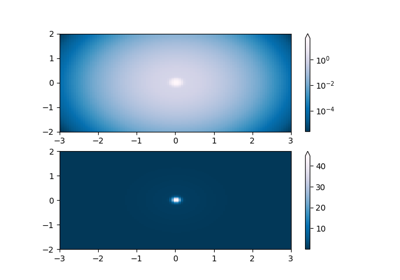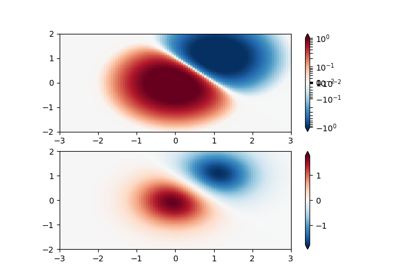# matplotlib.colors.SymLogNorm¶

class matplotlib.colors.SymLogNorm(linthresh, linscale=1.0, vmin=None, vmax=None, clip=False, *, base=None)[source]

The symmetrical logarithmic scale is logarithmic in both the positive and negative directions from the origin.

Since the values close to zero tend toward infinity, there is a need to have a range around zero that is linear. The parameter linthresh allows the user to specify the size of this range (-linthresh, linthresh).

Parameters: linthreshfloatThe range within which the plot is linear (to avoid having the plot go to infinity around zero). linscalefloat, default: 1This allows the linear range (-linthresh to linthresh) to be stretched relative to the logarithmic range. Its value is the number of powers of base to use for each half of the linear range. For example, when linscale == 1.0 (the default) and base=10, then space used for the positive and negative halves of the linear range will be equal to a decade in the logarithmic. basefloat, default: NoneIf not given, defaults to np.e (consistent with prior behavior) and warns. In v3.3 the default value will change to 10 to be consistent with SymLogNorm. To suppress the warning pass base as a keyword argument.
autoscale(self, A)[source]

Set vmin, vmax to min, max of A.

autoscale_None(self, A)[source]

Autoscale only None-valued vmin or vmax.

inverse(self, value)[source]

## Examples using matplotlib.colors.SymLogNorm¶